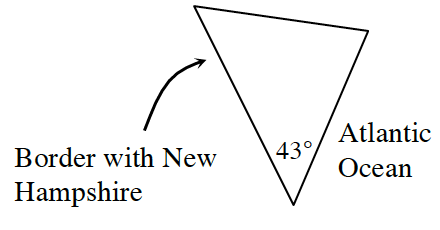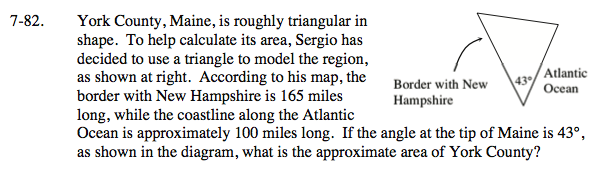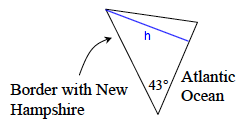Home > INT3 > Chapter 7 > Lesson 7.2.3 > Problem7-82

7-82.York County, Maine, is roughly triangular in shape. To help calculate its area, Sergio has decided to use a triangle to model the region, as shown at right. According to his map, the border with New Hampshire is 165 miles long, while the coastline along the Atlantic Ocean is approximately 100 miles long. If the angle at the tip of Maine is 43°, as shown in the diagram, what is the approximate area of York County? Homework Help ✎Find the height of the triangle.

$\text{sin}(43\degree)=\frac{\text{opp}}{\text{hyp}}=\frac{h}{165}$

h = 165 · sin (43°)

Then use this to find the area of the triangle:

$\frac{1}{2}(100)(165 \text{ sin}(43\degree))$Area ≈ 5626.5 square miles